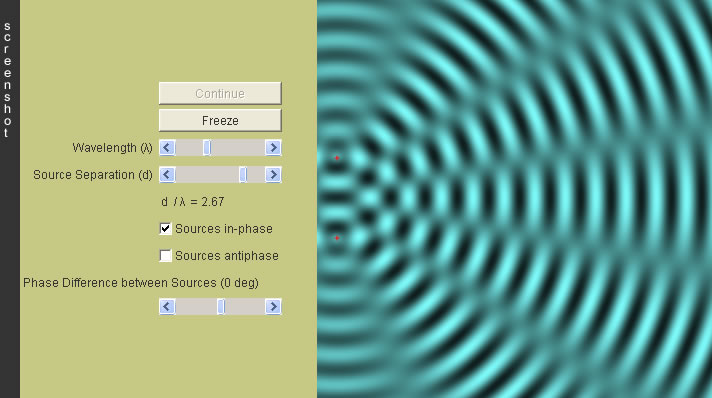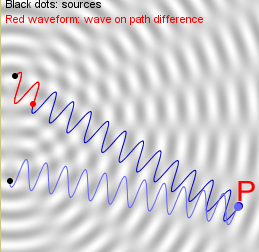Interference of Water Waves 1

 Internal Links : Phase difference Interference of water waves 2 Formation of beats (interference of two slightly different frequencies) Multiple Sources Interference (Grating)Simply speaking, the kind of interference taking place at a specific point, say P, is determined by the phase difference between the two sources (the two black dots), and the phase difference arising from the two unequal paths of travel of the two waves to that point. In the figure shown,the red waveform is the subtraction of the lower wave train from the upper one. After taking that, the two remaining parts (colored in blue) becomes equal in length. So, this red waveform is crucial and enough in knowing the phase difference of the second cause mentioned above.   Phase difference between the two waves arriving at P = Phase difference between the two sources (the two black dots) + Phase difference between the beginning and the end point of the red waveform
(I)

In particular, when the two sources are in-phase, then we only need to consider the effect from the path difference, which varies with the observation point P. As we know, wave repeats itself periodically over whole wavelengths. If the path difference (the red waveform) contains, e.g., 3 whole wavelengths, this means one of the two waves upon arrival at P will be shifted by 3 wavelengths more than the other; but importantly a shift of 3 wavelenths is equivalent to "zero shift". Since the two waves are in-phase as they are emanated, and such a path difference does not cause an additional nonzero phase shift, so they are still in-phase when they arrive at P. Hence, as the two waves meet and are added together there, a big up-down oscillation (constructive interference) is formed.

It is easy for us to conclude that:

• If the red waveform contains an integral number of wavelengths, the two waves arriving at P must be in phase, i.e.,
constructive interference occus at P when Path Difference = 0, λ, 2λ, 3λ, ....
• On the contrary, if the red waveform contains an integral number plus a half wavelength, the two waves arriving at P must be in anti-phase, i.e.,
destructive interference occurs at P when Path Difference = 0.5λ, 1.5λ, 2.5λ,..... , where λ is the wavelength.

(II) If the two sources are in anti-phase, the two waves are "exactly opposite" at the moment of leaving their sources.
A shift of a half wavelength because of the path difference will be just right to make them in-phase.
Therefore, the conditions now become
Constructive interference occurs at P when Path Difference = 0.5λ, 1.5λ, 2.5λ,..
Destructive interference occus at P when Path Difference = 0, λ, 2λ, 3λ, ....

(III) If the two sources have a phase difference, say π/3, what will the conditions of interference become?

Question:In the diagrams, P and Q are two points on the same interference pattern. The two sources are not necessarily in-phase. If the interference at P is destructive, what is the interference at Q ?The author (Chiu-king Ng) has the copyright on all the simulations in this website. Email phyAA@phy.hk, where AA is the prime number following 7. Last Update:2019-3-20